Test: Flow Through Pipes - 2

# Test: Flow Through Pipes - 2

Test Description

## 10 Questions MCQ Test GATE Civil Engineering (CE) 2023 Mock Test Series | Test: Flow Through Pipes - 2

Test: Flow Through Pipes - 2 for Mechanical Engineering 2022 is part of GATE Civil Engineering (CE) 2023 Mock Test Series preparation. The Test: Flow Through Pipes - 2 questions and answers have been prepared according to the Mechanical Engineering exam syllabus.The Test: Flow Through Pipes - 2 MCQs are made for Mechanical Engineering 2022 Exam. Find important definitions, questions, notes, meanings, examples, exercises, MCQs and online tests for Test: Flow Through Pipes - 2 below.
Solutions of Test: Flow Through Pipes - 2 questions in English are available as part of our GATE Civil Engineering (CE) 2023 Mock Test Series for Mechanical Engineering & Test: Flow Through Pipes - 2 solutions in Hindi for GATE Civil Engineering (CE) 2023 Mock Test Series course. Download more important topics, notes, lectures and mock test series for Mechanical Engineering Exam by signing up for free. Attempt Test: Flow Through Pipes - 2 | 10 questions in 30 minutes | Mock test for Mechanical Engineering preparation | Free important questions MCQ to study GATE Civil Engineering (CE) 2023 Mock Test Series for Mechanical Engineering Exam | Download free PDF with solutions
 1 Crore+ students have signed up on EduRev. Have you?
Test: Flow Through Pipes - 2 - Question 1

### Three identical pipes of length L, diameter D and friction factor f, are connected in parallel between two reservoirs. The size of a pipe of length L and of same friction factor f, equivalent to the above pipes, is

Detailed Solution for Test: Flow Through Pipes - 2 - Question 1

Equivalent pipe for the pipes in parallel: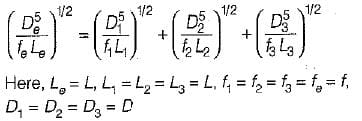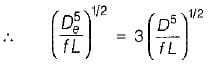or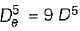or  De = 1.5518 D

Test: Flow Through Pipes - 2 - Question 2

### A pipe of diameter D is to be replaced by n pipe each of diameter of laid in parallel. The value of d is given by

Detailed Solution for Test: Flow Through Pipes - 2 - Question 2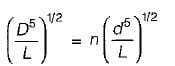or D5 = n2d5
or D = n2/5 D
∴ d = (D/n2/5)

Test: Flow Through Pipes - 2 - Question 3

### At a sudden expansion in a horizontal pipe

Test: Flow Through Pipes - 2 - Question 4

In a pipeline, the H.G.L is above the pipe center line in the longitudinal section at point A and below the pipe center line at another point B.
From this it can be inferred that

Test: Flow Through Pipes - 2 - Question 5

In network of pipes

Test: Flow Through Pipes - 2 - Question 6

Consider the following statements:
1. Pipe network analysis is normally necessary in analyzing flow in pipes at city water systems,
2. Hardy-cross method of solving pipe network is a method of successive approximations and is not a direct method.
3. The network must satisfy the momentum equation because the flow in each pipe satisfies the head loss equation.
4. Principle of continuity is satisfied in a pipe network..

Select the correct statments:

Test: Flow Through Pipes - 2 - Question 7

The diameter of the nozzle (d) for maximum transmission of power is given by
Where
D = Diameter of pipe
f = Darcy’s friction factor
l = Length of pipe

Detailed Solution for Test: Flow Through Pipes - 2 - Question 7

For maximum power transmission through nozzle:
Nozzle diameter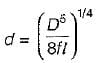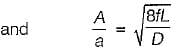A = area of supply pipe
a = area of Nozzle

Test: Flow Through Pipes - 2 - Question 8

Maximum efficiency of transmission of power through a pipe is

Detailed Solution for Test: Flow Through Pipes - 2 - Question 8

Efficiency of power transmission is given by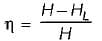For maximum efficiency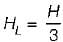We get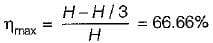Test: Flow Through Pipes - 2 - Question 9

Power transmitted through a pipe is maximum when
Where
HL = head loss due to friction

Detailed Solution for Test: Flow Through Pipes - 2 - Question 9

For maximum power transmission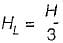Test: Flow Through Pipes - 2 - Question 10

Power transmitted through a pipe is given by
where
w = specific weight of the fluid flowing through pipe
Q = discharge, m3/s

Detailed Solution for Test: Flow Through Pipes - 2 - Question 10

Power transmitted through a pipe (When HL is frictional head)
P = wQ(H - HL)

## GATE Civil Engineering (CE) 2023 Mock Test Series

25 docs|282 tests
 Use Code STAYHOME200 and get INR 200 additional OFF Use Coupon Code
Information about Test: Flow Through Pipes - 2 Page
In this test you can find the Exam questions for Test: Flow Through Pipes - 2 solved & explained in the simplest way possible. Besides giving Questions and answers for Test: Flow Through Pipes - 2, EduRev gives you an ample number of Online tests for practice

## GATE Civil Engineering (CE) 2023 Mock Test Series

25 docs|282 tests